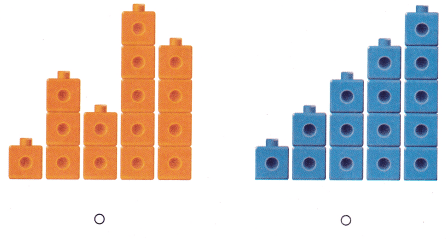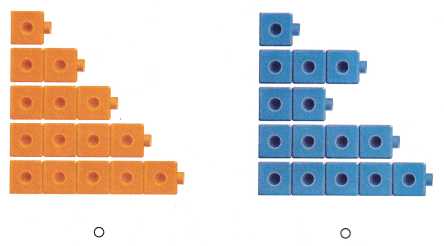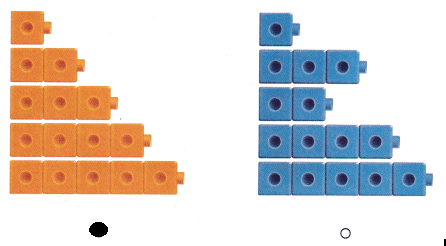# Texas Go Math Kindergarten Lesson 3.1 Answer Key Count and Order to 5

Refer to our Texas Go Math Kindergarten Answer Key Pdf to score good marks in the exams. Test yourself by practicing the problems from Texas Go Math Kindergarten Lesson 3.1 Answer Key Count and Order to 5.

## Texas Go Math Kindergarten Lesson 3.1 Answer Key Count and Order to 5

Explore

DIRECTIONS: Use cubes to make cube towers that have 1 to 5 cubes. Place the cube towers in order to match the numbers 1 to 5. Draw the cube towers in order.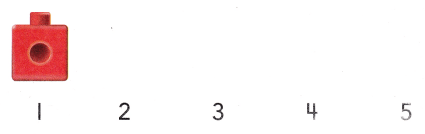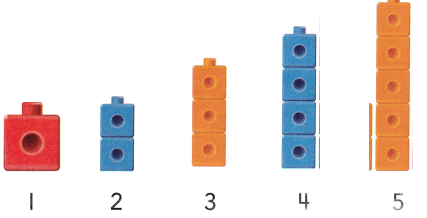Explanation:
Used cubes to make cube towers that have 1 to 5 cubes.
Placed the cube towers in order to match the numbers 1 to 5

Share and Show

DIRECTIONS: 1. Use cubes to make cube trains that have Ito 5 cubes. Place the cube trains in order beginning with 1. Draw the cube trains and write the numbers in order. Tell a friend what you know about the numbers and the cube trains.

Question 1.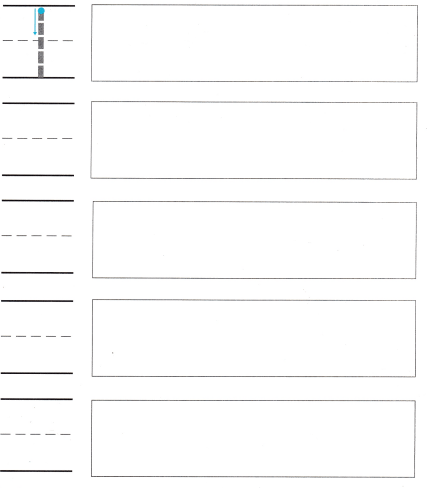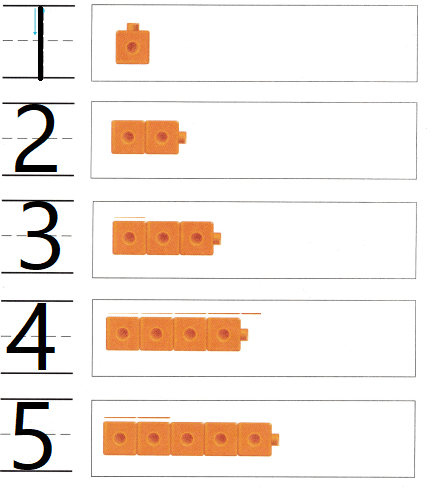Explanation:
Drawn cube train to match the number 1 to 5

DIRECTIONS: 2. Count the objects in each set. Write the number beside the set of objects. Write those numbers in order beginning with number 1.

Question 2.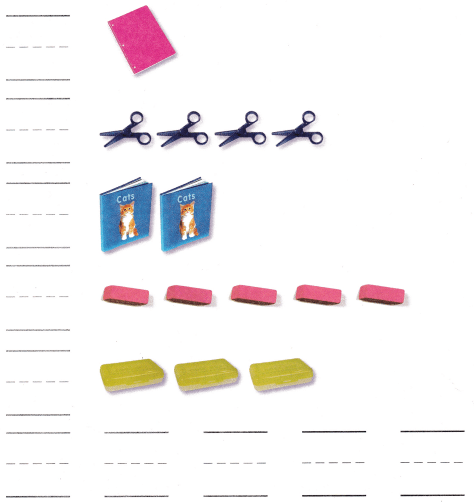Explanation:
Count the objects in each set.
Written the number beside the set of objects.
Written those numbers in order beginning with number 1.

HOME ACTIVITY • Show your child sets of objects from 1 to 5. Have him or her place the sets in order from 1 to 5.DIRECTIONS: 3. Which set of blocks shows a set that is one more than a set of 3 blocks? Circle that set of blocks. 4. Which cube trains are in counting order? Choose the correct answer.

Problem Solving

Question 3.Explanation:
The 4th set of blocks shows a set that is one more than a set of 3 blocks
and circle it.

Question 4.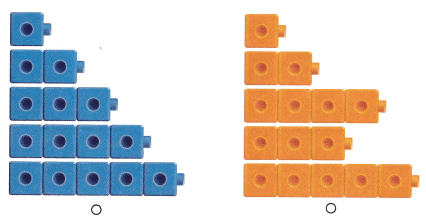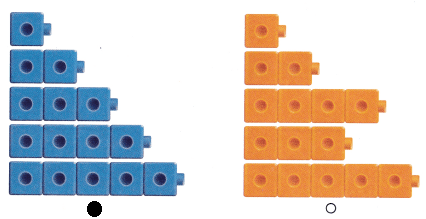Explanation:
The first set of cubes are in counting order
bubbled the correct option.

### Texas Go Math Kindergarten Lesson 3.1 Homework and Practice Answer Key

DIRECTIONS: 1. Count the objects in each set. Write the number beside the set of objects.

Question 1.Explanation:
Counted the objects in each set.
Written the number beside the set of objects

DIRECTIONS: Choose the correct answer. 2-3. Which cube trains ore in counting order?

Lesson Check

Question 2.